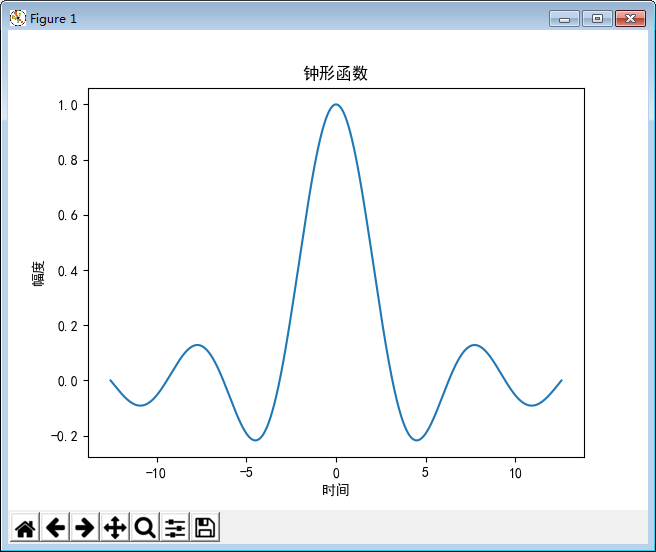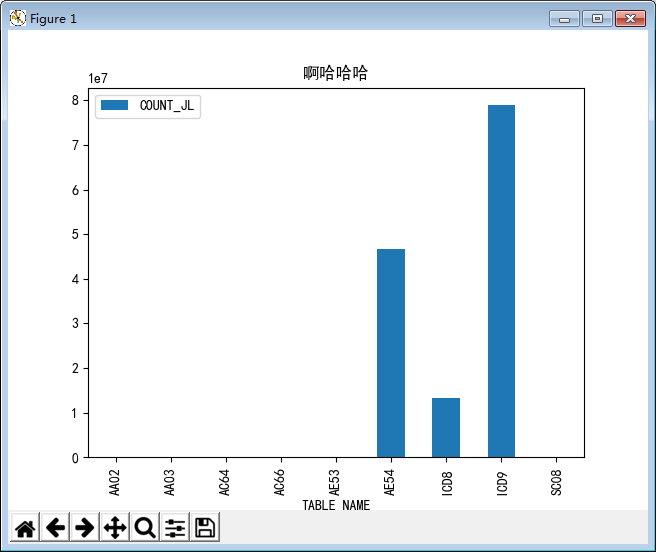• 每日干货好文分享丨请点击+关注欢迎关注天善智能微信公众号，我们是专注于商业智能BI，...在使用Python绘制图表前，我们需要先安装两个库文件numpy和matplotlib。Numpy是Python开源的数值计算扩展，可用来存储和处...

每日干货好文分享丨请点击+关注

欢迎关注天善智能微信公众号，我们是专注于商业智能BI，大数据，数据分析领域的垂直社区。

对商业智能BI、数据分析挖掘、大数据、机器学习，python，R感兴趣的加微信：tsbeidou，邀请你进入交流群，数据爱好者们都在这儿。

在使用Python绘制图表前，我们需要先安装两个库文件numpy和matplotlib。

Numpy是Python开源的数值计算扩展，可用来存储和处理大型矩阵，比Python自身数据结构要高效；matplotlib是一个Python的图像框架，使用其绘制出来的图形效果和MATLAB下绘制的图形类似。

下面我通过一些简单的代码介绍如何使用 Python绘图。

一、图形绘制直方图importmatplotlib.pyplotasplt

importnumpyasnp

mu=100

sigma=20

x=mu+sigma*np.random.randn(20000)# 样本数量

plt.hist(x,bins=100,color='green',normed=True)# bins显示有几个直方,normed是否对数据进行标准化

plt.show()

条形图importmatplotlib.pyplotasplt

importnumpyasnp

y=[20,10,30,25,15]

index=np.arange(5)

plt.bar(left=index,height=y,color='green',width=0.5)

plt.show()

折线图importmatplotlib.pyplotasplt

importnumpyasnp

x=np.linspace(-10,10,100)

y=x**3

plt.plot(x,y,linestyle='--',color='green',marker='<>

plt.show()

散点图importmatplotlib.pyplotasplt

importnumpyasnp

x=np.random.randn(1000)

y=x+np.random.randn(1000)*0.5

plt.scatter(x,y,s=5,marker='')#>

plt.show()

饼状图importmatplotlib.pyplotasplt

importnumpyasnp

labels='A','B','C','D'

fracs=[15,30,45,10]

plt.axes(aspect=1)#使x y轴比例相同

explode=[0,0.05,0,0]# 突出某一部分区域

plt.pie(x=fracs,labels=labels,autopct='%.0f%%',explode=explode)#autopct显示百分比

plt.show()

箱形图

主要用于显示数据的分散情况。图形分为上边缘、上四分位数、中位数、下四分位数、下边缘。外面的点时异常值importmatplotlib.pyplotasplt

importnumpyasnp

np.random.seed(100)

data=np.random.normal(size=(1000,4),loc=0,scale=1)

labels=['A','B','C','D']

plt.boxplot(data,labels=labels)

plt.show()

二、图像的调整

1、23种点形状'.'point','pixel'o'circle'v'triangle_down

'^'triangle_up''triangle_right'1'tri_down

'2'tri_up'3'tri_left'4'tri_right'8'octagon

's'square'p'pentagon'*'star'h'hexagon1'H'hexagon2

'+'plus'x'x'D'diamond'd'thin_diamond'triangle_left'>

2、8种內建默认颜色的缩写b:blueg:greenr:redc:cyan

m:magentay:yellowk:blackw:white

3、4种线性

- 实线 --虚线 -.点划线 ：点线

4、一张图上绘制子图importmatplotlib.pyplotasplt

importnumpyasnp

x=np.arange(1,100)

plt.subplot(221)#2行2列第1个图

plt.plot(x,x)

plt.subplot(222)

plt.plot(x,-x)

plt.subplot(223)

plt.plot(x,x*x)

plt.subplot(224)

plt.plot(x,np.log(x))

plt.show()

5、生成网格importmatplotlib.pyplotasplt

importnumpyasnp

y=np.arange(1,5)

plt.plot(y,y*2)

plt.grid(True,color='g',linestyle='--',linewidth='1')

plt.show()

6、生成图例importmatplotlib.pyplotasplt

importnumpyasnp

x=np.arange(1,11,1)

plt.plot(x,x*2)

plt.plot(x,x*3)

plt.plot(x,x*4)

plt.legend(['Normal','Fast','Faster'])

plt.show()

本文来源自天善社区博客，转载请保留以下内容：

本文作者：天善社区博客 超人Python

展开全文• 我正在用python做一些数据分析。我有一个时间序列数据(用于连续日期)，我想绘制如下内容：我matplotlib绘制了单线图，并使用base64编码将其嵌入到HTML中。但问题是我在HTML中嵌入图形时没有悬停功能。对于图像中...

我正在用python做一些数据分析。我有一个时间序列数据(用于连续日期)，我想绘制如下内容：我用matplotlib绘制了单线图，并使用base64编码将其嵌入到HTML中。但问题是我在HTML中嵌入图形时没有悬停功能。

对于图像中显示的图形类型，我可以使用matplotlib轻松地绘制它，但是为了便于阅读，我想将其嵌入html中并启用悬停功能。

我已经详细地看过了，这确实完成了工作，但图表并不是隐私的，隐私也是一个问题。在

为此，请推荐一些开源库。在

我所做的事情的代码：def graph(data):

keys = sorted(data.keys())

values = [data[key] for key in keys]

fig = plt.figure(figsize = (2 , 0.3) ,facecolor='w')

plt.axis("off")

plt.plot(values , color = 'g' )

imgdata = cStringIO.StringIO()

fig.savefig(imgdata, format='png' , facecolor = fig.get_facecolor())

s = '' %

imgdata.getvalue().encode("base64").strip()

plt.close()

return s

谢谢。在

展开全文• 尽管很卖力的写了中文要... plt.show()C:\Python27\lib\site-packages\matplotlib\font_manager.py:1297: UserWarning: findfont: Font family [u'sans-serif'] not found. Falling back to DejaVu Sans(prop.get...

尽管很卖力的写了中文要正确显示，

>>> plt.show()

C:\Python27\lib\site-packages\matplotlib\font_manager.py:1297: UserWarning: find

(prop.get_family(), self.defaultFamily[fontext]))

>>> plt.title(u'中文显示吧看好了')

>>> res[res['XH']>=28].groupby(res.TABLE_NAME).agg({'COUNT_JL':np.sum}).plot(kin

d='bar',title=u'中文你正常显示吧求你了！！')

但show()出来的结果差强人意。 努力的方式不对，这是智力上的懒惰。(你在一条漆黑的街道丢了钥匙，却在隔壁街道找寻，只因为隔壁街道灯光透亮。--《人件》)

网上查找了几种方法，比较推荐的一种是，每次使用都灵活设置一下字体编码

#-*- coding: utf-8 -*-

from pylab import *

mpl.rcParams['font.sans-serif'] = ['SimHei']

mpl.rcParams['axes.unicode_minus'] = False

t = arange(-4*pi, 4*pi, 0.01)

y = sin(t)/t

plt.plot(t, y)

plt.title(u'钟形函数')

plt.xlabel(u'时间')

plt.ylabel(u'幅度')

plt.show()

测试了一下，上面的办法可行。使用此办法。输入

>>> res[res['XH']>=28].groupby(res.TABLE_NAME).agg({'COUNT_JL':np.sum}).plot(kin

d='bar',title=u'啊哈哈哈')

plt.show()起到中文字符显示设定作用的代码

#-*- coding: utf-8 -*-

from pylab import *

mpl.rcParams['font.sans-serif'] = ['SimHei']

mpl.rcParams['axes.unicode_minus'] = False

展开全文• python对数据统计特别友好，原生库即有相关支持：matplotlib1.环境配置sudo pip install matplotlib安装完成后需要调整下配置文件(mac可跳过)，原因：python解释器各个平台版本都有，理论上会cover大多数底层接口，...

python对数据统计特别友好，原生库即有相关支持：matplotlib

1.环境配置sudo pip install matplotlib

安装完成后需要调整下配置文件(mac可跳过)，原因：python解释器各个平台版本都有，理论上会cover大多数底层接口，所以看起来“跨平台”。但是部分与绘图／进程／系统内核等相关功能，不同系统间差异较大，python尚未全cover。比如我们matplotlib在不同系统上兼容性就有点问题，所以需要通过配置文件来获得“跨平台”的效果。针对matplotlib有合适的egg包可以解决，修改配置文件让egg包生效即可。where is matlotlibrc

即可获得配置文件路径(windows请全盘搜索)，打开文件把backend改为以下Agg：# The default backend; one of GTK GTKAgg GTKCairo GTK3Agg GTK3Cairo

# CocoaAgg MacOSX Qt4Agg Qt5Agg TkAgg WX WXAgg Agg Cairo GDK PS PDF SVG

# Template.

# You can also deploy your own backend outside of matplotlib by

# referring to the module name (which must be in the PYTHONPATH) as

# ‘module://my_backend’.

backend : Agg

2.hello world# coding:utf-8

import matplotlib

matplotlib.use('Agg')

import matplotlib.pyplot as plt

import numpy as np

import os

plt.rcParams['font.sans-serif']=['SimHei']

plt.rcParams['axes.unicode_minus']=False

plt.plot( [12,34,56,78,90])#输入一个成员都是数字的数组

plt.savefig('tmp.png')#保存为图片

plt.close('all')#清空画布。不要省略这一句，不然多个图会有bug。

一共3句，一句都不能少，这就输出了个图片“tmp.png”hello world

3.定制坐标轴plt.plot( [12,34,56,78,90])

plt.xticks([0,1,2,3,4], ['1月'.decode('utf-8'),'2月'.decode('utf-8'),'3月'.decode('utf-8'),'4月'.decode('utf-8'),'5月'.decode('utf-8')], rotation=0)

plt.savefig('tmp.png')

plt.close('all')

以上只多了一行，输入2个数组，定制了x轴。第一个数组标记了文本出现的位置，第二个数组是unicode字符串。rotation可以让文字歪过来，在文本过长互相压盖时使用。定制坐标轴

4.定制图例plt.plot( [12,34,56,78,90],label='上月'.decode('utf-8'))

plt.legend(bbox_to_anchor=[0.3, 1])

plt.savefig('tmp.png')

plt.close('all')

画图时加个参数“label”，定义legend之后就会自动出图例。同样只支持unicode字符串。定制图例

5.定制标题plt.plot( [12,34,56,78,90])

plt.title('反馈率'.decode('utf-8'))

plt.savefig('tmp.png')

plt.close('all')

只需要加一句，传入unicode字符串。python默认是unicode，但是写了coding:utf-8之后会把默认编码修改成utf-8，所以这里需要decode回来。定制标题

6.定制图片尺寸fig=plt.gcf()

fig.set_size_inches(10, 5)

plt.plot( [12,34,56,78,90])

plt.savefig('tmp.png')

plt.close('all')

需要在绘制之前，设定图片尺寸，只需要2句。长宽单位是英寸，建议估摸着多试几次找满意的尺寸。定制图片尺寸

7.图表类型

常用的就 折线图，饼图，条形图；每种图有不同的定制方式。plt.plot([12,34,56,78,90],color='blue', label='反馈率'.decode('utf-8'),linewidth=5,linestyle='-.')

条形图比其他图多一个必选输入，需要输入条形长度和条形位置。

8.多子图

也就是在一张图片上，画多个图表。常见的就是上下组合，左右组合，4拼。不建议画更复杂的图。

左右：plt.figure(12)#先分成左右两块

plt.subplot(121)#绘制第一块

plt.plot([10,15,20])

plt.subplot(122)#绘制第2块

plt.plot([10,15,20])

plt.savefig('tmp.png')

plt.close('all')左右均等

上下：plt.figure(21)#先分成上下两块

plt.subplot(211)#绘制第一块

plt.plot([10,15,20])

plt.subplot(212)#绘制第2块

plt.plot([10,15,20])

plt.savefig('tmp.png')

plt.close('all')上下均等plt.figure(22)#先分成4两块

plt.subplot(221)#绘制第一块

plt.plot([10,15,20])

plt.subplot(222)#绘制第2块

plt.plot([10,15,20])

plt.subplot(223)#绘制第3块

plt.plot([10,15,20])

plt.subplot(224)#绘制第4块

plt.plot([10,15,20])

plt.savefig('tmp.png')

plt.close('all')4拼

展开全文• 本篇文章小编给大家分享一下Python绘制组合图代码示例，文章代码介绍的很详细，小编觉得挺不错的，现在分享给大家供大家参考，有需要的小伙伴们可以来看看。绘制组合图：组合图就是将多个形状，组合到⼀个图形中，...
• 一、绘制圆环图import numpy as npimport matplotlib.pyplot as plt#解决中文乱码问题plt.rcParams['font.sans-serif'] = ['Arial Unicode MS']# 解决负号无法正常显示的问题plt.rcParams['axes.unicode_minus']=...
• Python绘制图表

2021-01-29 13:43:46
Python 绘制图表import matplotlib.pyplot as pltimport numpy as npMatplotlib模块X=np.linspace(start = -np.pi, stop=np.pi, num=256, endpoint=True)C,S = np.cos(X), np.sin(X)plt.plot(X, C)plt.plot(X, S)plt...python制图表
• 条形图在数据可视化里，是一个经常被使用到的图表。虽然很好，也还是存在着缺陷。比如条形图条目太多时，会显得臃肿，不够直观。棒棒糖图表则是对条形图的改进，以一种小清新的设计，清晰明了表达了我...数据可视化 可视化 html scala go
• 大家好，我是涛哥条形图在数据可视化里，是一个经常被使用到的图表。虽然很好，也还是存在着缺陷呢。比如条形图条目太多时，会显得臃肿，不够直观。棒棒糖图表则是对条形图的改进，以一种小清新的设计...python 数据可视化 可视化 数据分析 canvas
• 也许你会认为python不适合做图形界面的开发，的确如此，但是python却有一个非常漂亮的图标模块：pycha，废话少说，先上图，各位看一下。 是不是效果还不错呢，当然这只是一小部分图表，还有其他很多的例子。下面我来...
• python图表制作

2021-02-10 04:19:19
画一个吸引人注意的图表相当重要。当你探索一个数据集，需要画图表图表看起来令人愉悦是件很高兴的事。在与你的观众交流观点时，可视化同样重要，同时，也很有必要去让图表吸引注意力和印入...我们ipython no...
• 代码 ''' Author: CloudSir Date: 2021-07-28 10:...Description: python绘制3D图表 https://github.com/cloudsir ''' from mpl_toolkits.mplot3d import Axes3D import numpy as np from matplotlib import pyplot aspython 数据可视化
• 将鼠标悬停在图表上时，将弹出注释· 交互性：无需任何其他设置即可使图表互动(例如，穿越时空的旅程)· 漂亮的地理空间图：Plotly具有一些内置的基本地图绘制功能，但是另外，可以使用mapbox集成来生成惊人的图表。...
• 使用Python绘制一幅专业的K线图，是量化投资和金融数据分析的必备功课。 下面我将从K线图简介、数据获取、K线图绘制及成交量绘制等方面，结合源代码，一步步实现专业K线图的绘制。 K线图简介 K线图又被成为“蜡烛图...python
• 对于初学者来说，很容易被这官网上众多的图表类型给吓着了，由于种类太多，几种图表绘制方法很有可能会混淆起来。 因此，在这里，我特地总结了六种常见的基本图表类型，你可以通过对比学习，打下坚实的基础。 ...python 编程语言 数据可视化 可视化 数据分析
• 下面我就给大家介绍一下，如何使用Python绘制棒棒糖图表使用到的是我国1949到2019年，历年的出生人口数据，数据来源国家统计局。 首先读取一下数据。 importpandasaspd importmatplotlib.pyp...
• 使用python绘制中国地图

千次阅读 2020-12-30 07:22:23
为了实现将数据可视化与地图相结合，我们使用python中的一个第三方模块pyecharts，这个模块的原理就是python调用echarts的功能生成相关的图表文档。本文从以下三个方面展开讲解pyecharts环境的安装中国地图的绘制关....python绘制中国
• 序言：Python的可视化工具,以下截图，均以展示图表实例，如需了解部分对象的输出结果，可参照我Github上的代码，3Q????【课程3.13】 表格样式创建表格视觉样式：Dataframe.style → 返回pandas.Styler对象的属性，...
• 其实这些问题看看下面的实例图形就已不攻自破了，地理图表一看首先就是地图，然后在地理图表里面展示数据，比如说热力图，趋势流动图，人口密集分布图，反正地理坐标相关的就可以运用在这个里面，其次图形支持全球...数据可视化 热力图 地图 pyecharts Python画图
• 本文利用Python绘制简单的散点图，需使用Matplotlib安装包，该安装包的安装可参考： Python中安装Matplotlib数学绘图库_Xx_Studying的博客-CSDN博客 绘制简单的散点图详细的python代码： import matplotlib....python 开发语言 后端 数据可视化
• 图表是比干巴巴的表格更直观的表达，简洁、有力。工作中经常遇到的场景是，有一些...把数值转化为图片要依赖第三方库的帮忙，在Python之中最好的图表库叫matplotlib。(一直觉得，Python最大的优势就是丰富的第三方...
• 本文利用Python绘制简单的折线图，需使用Matplotlib安装包，该安装包的安装可参考： Python中安装Matplotlib数学绘图库_Xx_Studying的博客-CSDN博客 绘制简单的折线图详细的python代码： import matplotlib....python 开发语言 数据可视化
• Python使用matplotlib可视化绘制并导出可视化结果图表到PDF文件中 #仿真数据1 from pandas import DataFrame Data1 = {'Unemployment_Rate': [6.1,5.8,5.7,5.7,5.8,5.6,5.5,5.3,5.2,5.2], 'Stock_Index_Price...python 数据挖掘 机器学习 人工智能 自然语言处理
• 原标题：如何用Python绘制学术报告图表？作者：ExcelTing原文：http://cnblogs.com/excelting/p/6507680.html全文约 3581 字，读完可能需要 5 分钟。论文中图表形式多样，常用的处理工具有excel、MATLAB以及Python等...
• 我发现了一张有趣的图表，它...在我想用python重现这种艺术。在现在，我可以使用Plotly包以相同的样式打印表。在import plotly.plotly as pyimport plotly.graph_objs as gofrom plotly.tools import FigureFactory...
• 拖拽之间就能用Python的Matplotlib、Seaborn做可视化了～python 数据分析 数据可视化
• 使用Python绘制股票交易图形

千次阅读 2021-05-25 16:16:30
使用Python绘制股票交易图形使用Python绘制股票交易图形关联知识点准备工作数据载入任务一：绘制2020年1月1日至2020年12月31日的价格走势任务二：绘制2020年1月至2020年12月的成交量柱状图任务三：绘制2020年1月至...数据分析 python 数据可视化
• 离散型数据可视化饼图#以失信用户数据为例，绘制学历的分布饼图import matplotlib.pyplot as pltedu = [0.2515,0.3724,0.3336,0.0368,0.0057]labels = ['中专','大专','本科','硕士','其他']#生成数据，用于突出显示......

用python绘制图表代码python 订阅